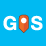# True/False Statement for a definition

3401
6
04-09-2015 10:06 AMNew Contributor II

Hi All,

I am trying to create a True Statement to check if a definition passed the "if"

On line 19 i am running "DEF RENAME" (Changes the name so the tool will run and I can fix it later)

Line 20 I want to know if Rename passed the if statement

My code is to change folders and shapefiles into a GDB while maintaining the structure of the folders.  Please let me know if you have advice or see a large issue.  The way I run this is through the command line.

`def rename(variable):    desc = arcpy.Describe(variable)    name = desc.name    new_name = "renamed"    print name    if " " or "-" or "0" or "1" or "2" or "3" or "4" or "5" or "6" or "7" or "8" or "9" in name:        print("{0} is being changed to: {1}".format(name, new_name))        arcpy.Rename_management(variable, new_name)    else:        passdef import_to_GDB(input_workspace, output_workspace):    import os    base = os.path.basename(input_workspace)    arcpy.CreateFileGDB_management(output_workspace, base)    for dirpath, dirnames, filenames in arcpy.da.Walk(input_workspace):        for dirname in dirnames:            try:                test = rename(dirname)                if test == True:                    dirname = "renamed"                    dir_path = os.path.join(dirpath, dirname)                    arcpy.env.workspace = dir_path                    fcs = arcpy.ListFeatureClasses()                    fds = os.path.join(output_workspace, base + ".gdb\\")                    arcpy.CreateFeatureDataset_management(fds, dirname)                    suboutput = os.path.join(fds, dirname)                    count = 0                else:                    dir_path = os.path.join(dirpath, dirname)                    arcpy.env.workspace = dir_path                    fcs = arcpy.ListFeatureClasses()                    fds = os.path.join(output_workspace, base + ".gdb\\")                    arcpy.CreateFeatureDataset_management(fds, dirname)                    suboutput = os.path.join(fds, dirname)                    count = 0            except:                print("{0} had an error, passing to the next object".format(dirname))            for fc in fcs:                count = count + 1                try:                    arcpy.FeatureClassToGeodatabase_conversion(fc, suboutput)                    print("{0} Complete".format(count))                except:                    print("{0} had an error, passing to the next object".format(fc))`
6 RepliesbyMVP Regular Contributor

Are you having an issue running this code or are you just looking for suggestions to improve your code that's already working?New Contributor II

Maybe it can't describe the name of a folder?

Sorry I wasn't clear, it is blowing up on me... here is the error:

Runtime error

Traceback (most recent call last):

File "<string>", line 1, in <module>

File "<string>", line 26, in import_to_GDB

File "<string>", line 2, in rename

File "c:\program files (x86)\arcgis\desktop10.1\arcpy\arcpy\__init__.py", line 1200, in Describe

return gp.describe(value)

File "c:\program files (x86)\arcgis\desktop10.1\arcpy\arcpy\geoprocessing\_base.py", line 374, in describe

self._gp.Describe(*gp_fixargs(args, True)))

IOError: "Buffer" does not existbyMVP Esteemed Contributor

This will give you some ideas

`>>> name = 'bob1'>>> nums = '0123456789- '>>> vals = [i for i in nums]>>> vals['0', '1', '2', '3', '4', '5', '6', '7', '8', '9', '-', ' ']>>> for i in vals:...  print i, i in name... 0 False1 True2 False3 False4 False5 False6 False7 False8 False9 False- False  False>>>`New Contributor II

Dan,

Thanks alot you got the ball rolling for some interesting thingsNew Contributor II
`def import_to_GDB(input_workspace, output_workspace):    import arcpy    import os    base = os.path.basename(input_workspace)    arcpy.CreateFileGDB_management(output_workspace, base)    for dirpath, dirnames, filenames in arcpy.da.Walk(input_workspace):        for dirname in dirnames:            try:                x = os.path.join(dirpath, dirname)                desc = arcpy.Describe(x)                name = desc.name                print("Working on: {0}".format(name))                if "-" in name:                    new_name = "rename"                    arcpy.Rename_management(x, new_name)                    dir_path = os.path.join(dirpath, new_name)                    arcpy.env.workspace = dir_path                    fcs = arcpy.ListFeatureClasses()                    fds = os.path.join(output_workspace, base + ".gdb\\")                    arcpy.CreateFeatureDataset_management(fds, new_name)                    suboutput = os.path.join(fds, new_name)                    count = 0                    for fc in fcs:                        count = count + 1                        try:                            arcpy.FeatureClassToGeodatabase_conversion(fc, suboutput)                            print("{0} Complete".format(count))                        except:                            print("{0} had an error, passing to the next object".format(fc))                else:                    dir_path = os.path.join(dirpath, dirname)                    arcpy.env.workspace = dir_path                    fcs = arcpy.ListFeatureClasses()                    fds = os.path.join(output_workspace, base + ".gdb\\")                    arcpy.CreateFeatureDataset_management(fds, dirname)                    suboutput = os.path.join(fds, dirname)                    count = 0                    for fc in fcs:                        count = count + 1                        try:                            arcpy.FeatureClassToGeodatabase_conversion(fc, suboutput)                            print("{0} Complete".format(count))                        except:                            print("{0} had an error, passing to the next object".format(fc))            except:                print("{0} had an error, passing to the next object".format(dirname))`

This currently works, but it only tests for a "-"  it was passing the if statment everytime if i tested " " .... any ideas?byMVP Honored Contributor

You could make a list of things to check (checklist below), then find the matches between it and the name:

`>>> name = 'john doe1'>>> checklist = [' ','-','1','2','3','4']>>> matches = [i for i in checklist if i in name]>>> matches[' ', '1']`